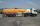# Quadrangular prism

The quadrangular prism has a volume 648 cm3. Trapezoid which is its base has the dimensions bases: a = 10 cm, c = 5 and height v = 6 cm. What is the height of the prism?

Result

h =  14.4 cm

#### Solution:Leave us a comment of example and its solution (i.e. if it is still somewhat unclear...):

Showing 0 comments:Be the first to comment!#### To solve this example are needed these knowledge from mathematics:

Tip: Our volume units converter will help you with converion of volume units.

## Next similar examples:

1. Square prismCalculate the volume of a foursided prism 2 dm high, the base is a trapezoid with bases 12 cm, 6 cm, height of 4 cm and 5 cm long arms.
2. TrenchThe trench is a four-sided prism. The cross section has a trapezoidal shape with basements of 4m and 6m, the length of the trench is 30m. What is the depth of the trench if we dig 60,000 l of soil.
3. Triangular prismCalculate the volume of a triangular prism 10 cm high, the base of which is an equilateral triangle with dimensions a = 5 cm and height va = 4,3 cm
4. Triangular prismCalculate the surface area and volume of a triangular prism, base right triangle if a = 3 cm, b = 4 cm, c = 5 cm and height of prism h=12 cm.
5. Scale factorA prism with a volume of 1458 mm3 is scaled down to a volume of 16mm3. What is the scale factor in fraction form?
6. Gasoline tank cylindricalWhat is the inner diameter of the tank, which is 8 m long and contains 40 cubic cubic meters of gasoline?
7. The cylinder baseThe cylinder with a base of 8 dm2 has a volume of 120 liters. From a cylinder fully filled with water, 40 liters of water was removed. At what height from the bottom /with precision to dm/ is the water level?
8. Cuboid enlargementBy how many percent increases the volume of cuboid if its every dimension increases by 30%?
9. KLMN trapezoidThe KLMN trapezoid has bases KL 40cm and MN 16cm. On the KL base is point P. The segment NP divides the trapezoid into units with the same area. What is the distance of point P from point K?
10. Roof tilesThe roof has a trapezoidal shape with bases of 15 m and 10 m, height of roof is 4 meters. How many tiles will need if on 1 m2 should be used 8 tiles?
11. TrapeziumThe area of trapezium is 35 cm2. Find its altitude if the bases are 6cm and 8 cm.
12. Trapezoid MO-5-Z8Trapezoid KLMN has bases 12 and 4 cm long. The area of triangle KMN is 9 cm2. What is the area of the trapezoid KLMN?
13. Trapezium 2Trapezium has an area of 24 square cms. How many different trapeziums can be formed ?
14. Holidays - on poolChildren's tickets to the swimming pool stands x € for an adult is € 2 more expensive. There was m children in the swimming pool and adults three times less. How many euros make treasurer for pool entry?
15. AceThe length of segment AB is 24 cm and the point M and N divided it into thirds. Calculate the circumference and area of this shape.
16. Circular lawnAround a circular lawn area is 2 m wide sidewalk. The outer edge of the sidewalk is curb whose width is 2 m. Curbstone and the inner side of the sidewalk together form a concentric circles. Calculate the area of the circular lawn and the result round to 1
17. Percentages52 is what percent of 93?